# 栈和队列的数据结构的实现过程（Java 实现）

## 栈的数据结构的实现

### 栈的基础知识回顾

1. 栈是一种线性结构

2. 相比数组，栈对应的操作是数组的子集。

• 也就是栈可基于数组实现，可以将栈看成一种特殊的数组。
3. 栈只能从一端添加元素，也只能从一端取出元素，这一端称为栈顶

4. 栈是一种 后进先出 (LIFO: Last In First Out) 的数据结构。

### 栈的常见应用

• 撤销（Undo）操作• 程序调用的系统栈### 基于数组的栈的实现

1. 入栈：void push(E element)2. 出栈: E pop()3. 查看栈顶元素: E peek()4. 获取栈中元素个数: int getSize()5. 判断栈是否为空: boolean: isEmpty()#### 具体代码设计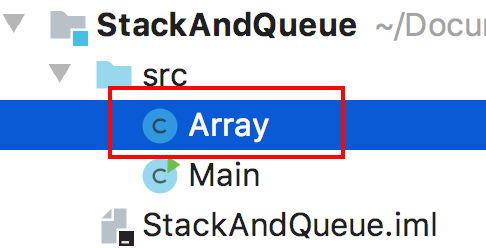ArrayStack 类的实现:

/**
* 获取数组的最后一个元素
*
* @return 返回数组的最后一个元素
*/
public E getLast() {
return get(size - 1);
}

/**
* 获取数组的第一个元素
*
* @return 返回数组的第一个元素
*/
public E getFirst() {
return get(0);
}


Stack 接口类代码如下:

/**
* 定义栈的基本操作的接口
* 支持泛型
*
* @author 踏雪寻梅
* @date 2020/1/8 - 19:20
*/
public interface Stack<E> {
/**
* 获取栈中元素个数
*
* @return 栈中如果有元素,返回栈中当前元素个数;栈中如果没有元素返回 0
*/
int getSize();

/**
* 判断栈是否为空
*
* @return 栈为空,返回 true;栈不为空,返回 false
*/
boolean isEmpty();

/**
* 入栈
* 将元素 element 压入栈顶
*
* @param element 入栈的元素
*/
void push(E element);

/**
* 出栈
* 将当前栈顶元素出栈并返回
*
* @return 返回当前出栈的栈顶元素
*/
E pop();

/**
* 查看当前栈顶元素
*
* @return 返回当前的栈顶元素
*/
E peek();
}


ArrayStack 类代码实现如下：

/**
* 基于之前实现的动态数组类 Array 实现的数组栈类 ArrayStack
* 同样支持泛型
*
* @author 踏雪寻梅
* @date 2020/1/8 - 19:26
*/
public class ArrayStack<E> implements Stack<E> {
/**
* 动态数组 array
* 基于 array 实现栈的操作
*/
private Array<E> array;

/**
* 构造函数
* 创建一个容量为 capacity 的数组栈
*
* @param capacity 要创建的栈的容量,由用户指定
*/
public ArrayStack(int capacity) {
array = new Array<>(capacity);
}

/**
* 默认构造函数
* 创建一个默认容量的数组栈
*/
public ArrayStack() {
array = new Array<>();
}

@Override
public int getSize() {
// 复用 array 的 getSize() 方法即可
return array.getSize();
}

@Override
public boolean isEmpty() {
// 复用 array 的 isEmpty() 方法即可
return array.isEmpty();
}

/**
* 获取栈的容量
* ArrayStack 特有的方法
*
* @return 返回栈的容量
*/
public int getCapacity() {
// 复用 array 的 getCapacity() 方法即可
return array.getCapacity();
}

@Override
public void push(E element) {
// 将数组的末尾作为栈顶,复用 array 的 addLast() 方法实现
}

@Override
public E pop() {
// 将数组的末尾作为栈顶,复用 array 的 removeLast() 方法将栈顶元素出栈并返回
return array.removeLast();
}

@Override
public E peek() {
// 将数组的末尾作为栈顶,复用 array 的 getLast() 方法获取栈顶元素
return array.getLast();
}

/**
* 重写 toString 方法返回数组栈的信息
*
* @return 返回数组栈的当前信息
*/
@Override
public String toString() {
StringBuilder result = new StringBuilder();
result.append("ArrayStack: ");
result.append("size: ").append(array.getSize()).append(" ");
result.append("capacity: ").append(array.getCapacity()).append(" ");
result.append("bottom -> [ ");
for (int i = 0; i < array.getSize(); i++) {
result.append(array.get(i));
// 如果不是最后一个元素
if (i != array.getSize() - 1) {
result.append(", ");
}
}
result.append(" ] <- top");
return result.toString();
}
}


/**
* 测试 ArrayStack
*
* @author 踏雪寻梅
* @date 2020/1/8 - 16:49
*/
public class Main {
public static void main(String[] args) {
ArrayStack<Integer> stack = new ArrayStack<>();

for (int i = 0; i < 10; i++) {
// 入栈
stack.push(i);
// 打印入栈过程
System.out.println(stack);
}

// 进行一次出栈
stack.pop();
// 查看出栈后的状态
System.out.println(stack);

// 查看当前栈顶元素
Integer topElement = stack.peek();
System.out.println("当前栈顶元素： " + topElement);

// 判断栈是否为空
System.out.println("当前栈是否为空: " + stack.isEmpty());
}
}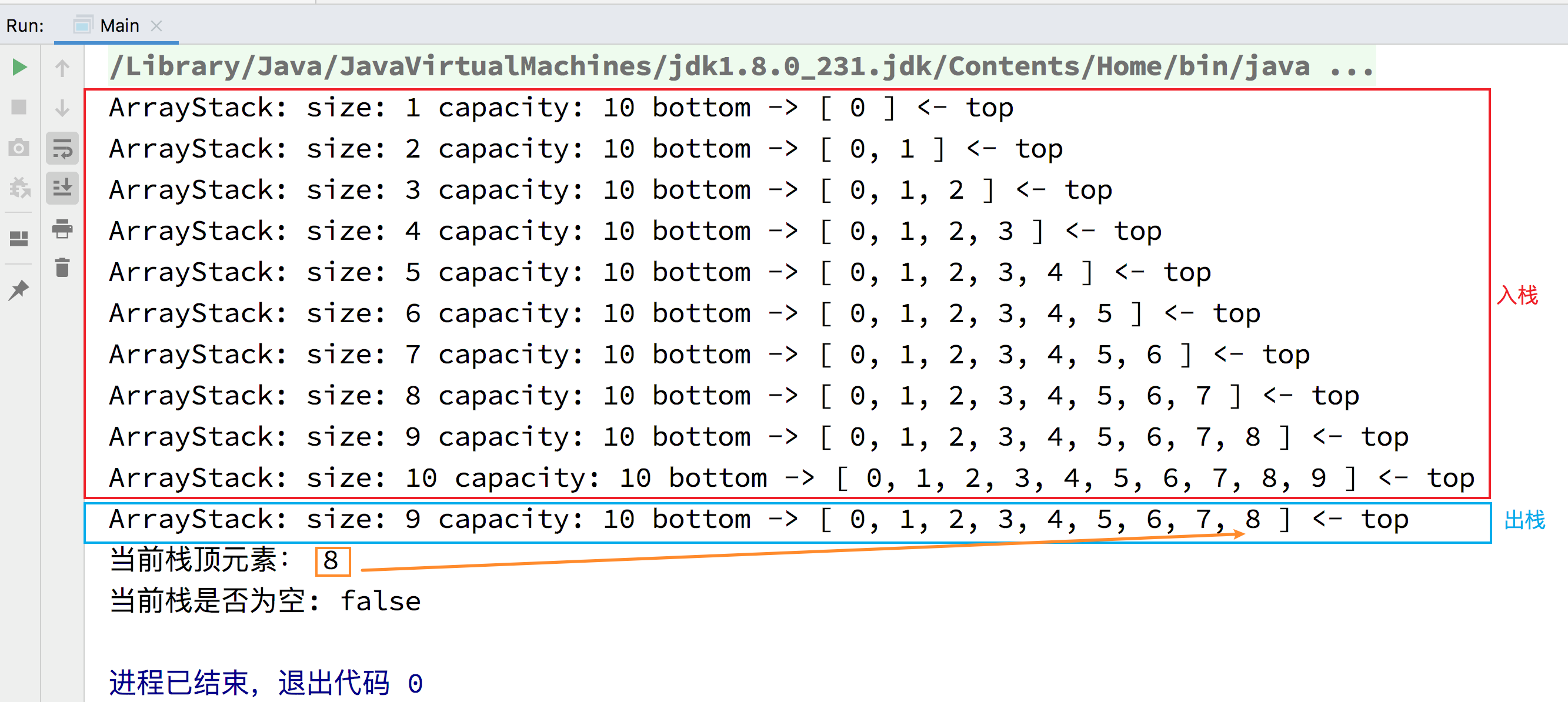#### 基于数组的栈简单的时间复杂度分析

• void push(E element)：O(1) 均摊

• 复用了 array 的方法，所以可能触发 resize 方法进行伸缩容量，所以时间复杂度是均摊的。
• E pop()：O(1) 均摊

• 复用了 array 的方法，所以可能触发 resize 方法进行伸缩容量，所以时间复杂度是均摊的。
• E peek()：O(1)

• int getSize()：O(1)

• boolean isEmpty()：O(1)

### 关于栈的一个算法应用：括号匹配

给定一个只包括 '('，')'，'{'，'}'，'['，']' 的字符串，判断字符串是否有效。

左括号必须用相同类型的右括号闭合。
左括号必须以正确的顺序闭合。
注意空字符串可被认为是有效字符串。

输入: "()"
输出: true

输入: "()[]{}"
输出: true

输入: "(]"
输出: false

输入: "([)]"
输出: false

输入: "{[]}"
输出: true



• 逐一遍历给定字符串中的括号。
• 如果括号是一个左括号，就将其压入栈中。

• 如果遇到右括号，此时将栈顶的左括号出栈查看是否可以和这个右括号匹配。

• 如果可以匹配，则继续剩余的判断。

• 如果所有括号匹配成功后，那么此时栈应该是空栈，说明给定字符串是有效的。
• 如果不可以匹配，则说明这个字符串是无效的。

• 有效的括号字符串图示• 无效的括号字符串图示• 方法一: 使用 java 的 util 包中内置的 Stack 类解决。

import java.util.Stack;

/**
* 括号匹配解决方案
* 方法一：使用 java 的 util 包中内置的 Stack 类解决
*
* @author 踏雪寻梅
* @date 2020/1/8 - 21:52
*/
public class Solution {
public boolean isValid(String s) {
Stack<Character> stack = new Stack<>();

// 遍历给定字符串
for (int i = 0; i < s.length(); i++) {
// 查看括号
char c = s.charAt(i);
// 如果括号是左括号,入栈
if (c == '(' || c == '[' || c == '{') {
stack.push(c);
} else {
// 括号是右括号,查看是否和栈顶括号相匹配
if (stack.isEmpty()) {
// 如果此时栈是空的,说明前面没有左括号,字符串是右括号开头的,匹配失败,字符串无效
return false;
}

// 栈非空,将当前栈顶括号出栈保存到变量中进行匹配判断
char topBracket = stack.pop();
// 匹配失败的情况,以下情况若为发生则进行下一次循环依次判断
if (c == ')' && topBracket != '(') {
return false;
}
if (c == ']' && topBracket != '[') {
return false;
}
if (c == '}' && topBracket != '{') {
return false;
}
}
}

// for 循环结束后,如果栈中还有字符,说明有剩余的左括号未匹配,此时字符串无效,否则字符串有效
// 即 isEmpty() 返回 true 表示匹配成功;返回 false 表示匹配失败
return stack.isEmpty();
}
}

• 提交结果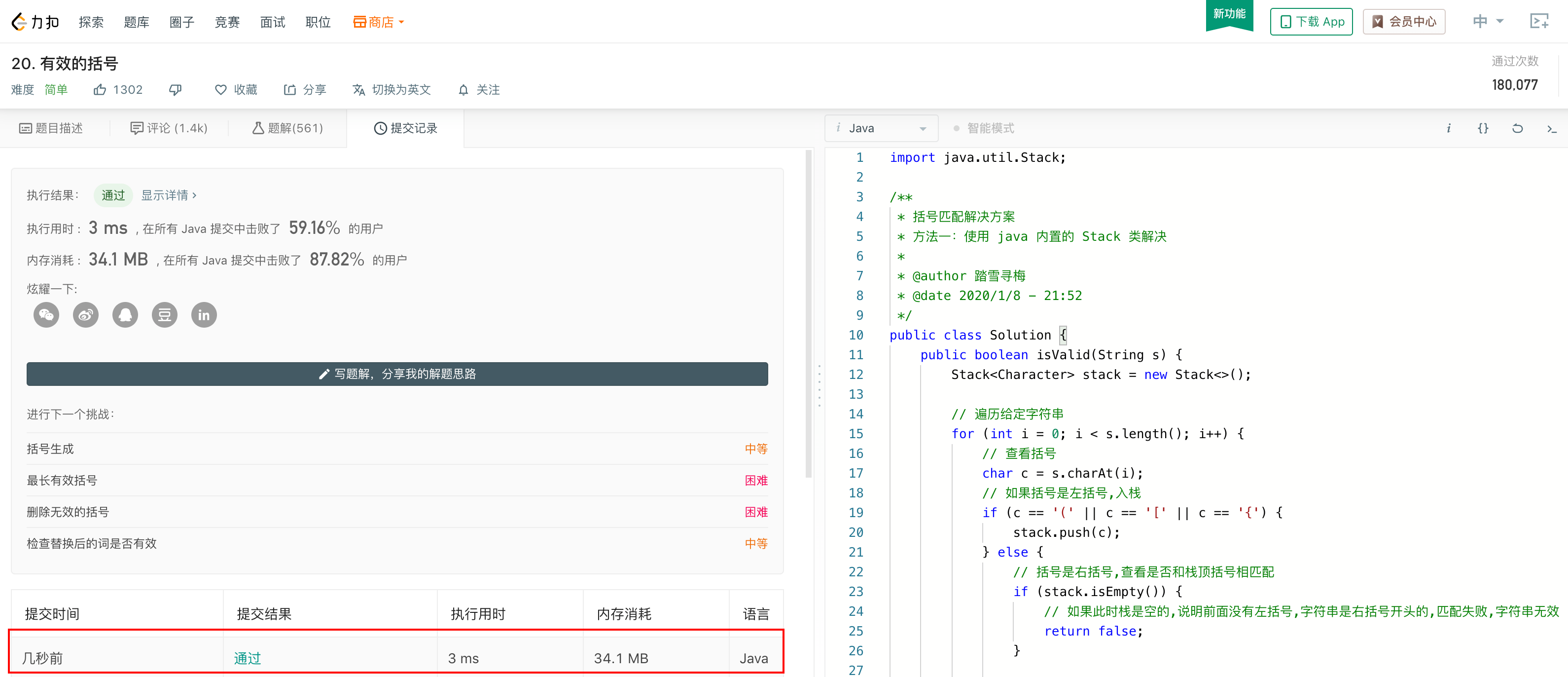• 方法二：使用自己实现的 ArrayStack 类解决

需要注意的是要在 Solution 中添加自己实现的 Array 类、Stack 接口、ArrayStack 类作为内部类才能使用自己实现的数组栈来解决。

/**
* 括号匹配解决方案
* 方法二：使用自己实现的 ArrayStack 类解决
*
* @author 踏雪寻梅
* @date 2020/1/8 - 22:25
*/
public class Solution {
public boolean isValid(String s) {
Stack<Character> stack = new ArrayStack<>();

// 遍历给定字符串
for (int i = 0; i < s.length(); i++) {
// 查看括号
char c = s.charAt(i);
// 如果括号是左括号,入栈
if (c == '(' || c == '[' || c == '{') {
stack.push(c);
} else {
// 括号是右括号,查看是否和栈顶括号相匹配
if (stack.isEmpty()) {
// 如果此时栈是空的,说明前面没有左括号,字符串是右括号开头的,匹配失败,字符串无效
return false;
}

// 栈非空,将当前栈顶括号出栈保存到变量中进行匹配判断
char topBracket = stack.pop();
// 匹配失败的情况,以下情况若为发生则进行下一次循环依次判断
if (c == ')' && topBracket != '(') {
return false;
}
if (c == ']' && topBracket != '[') {
return false;
}
if (c == '}' && topBracket != '{') {
return false;
}
}
}

// for 循环结束后,如果栈中还有字符,说明有剩余的左括号未匹配,此时字符串无效,否则字符串有效
// 即 isEmpty() 返回 true 表示匹配成功;返回 false 表示匹配失败
return stack.isEmpty();
}

private class Array<E> {
...（该类中的代码此处省略）
}

private interface Stack<E> {
...（该类中的代码此处省略）
}

private class ArrayStack<E> implements Stack<E> {
...（该类中的代码此处省略）
}
}

• 提交结果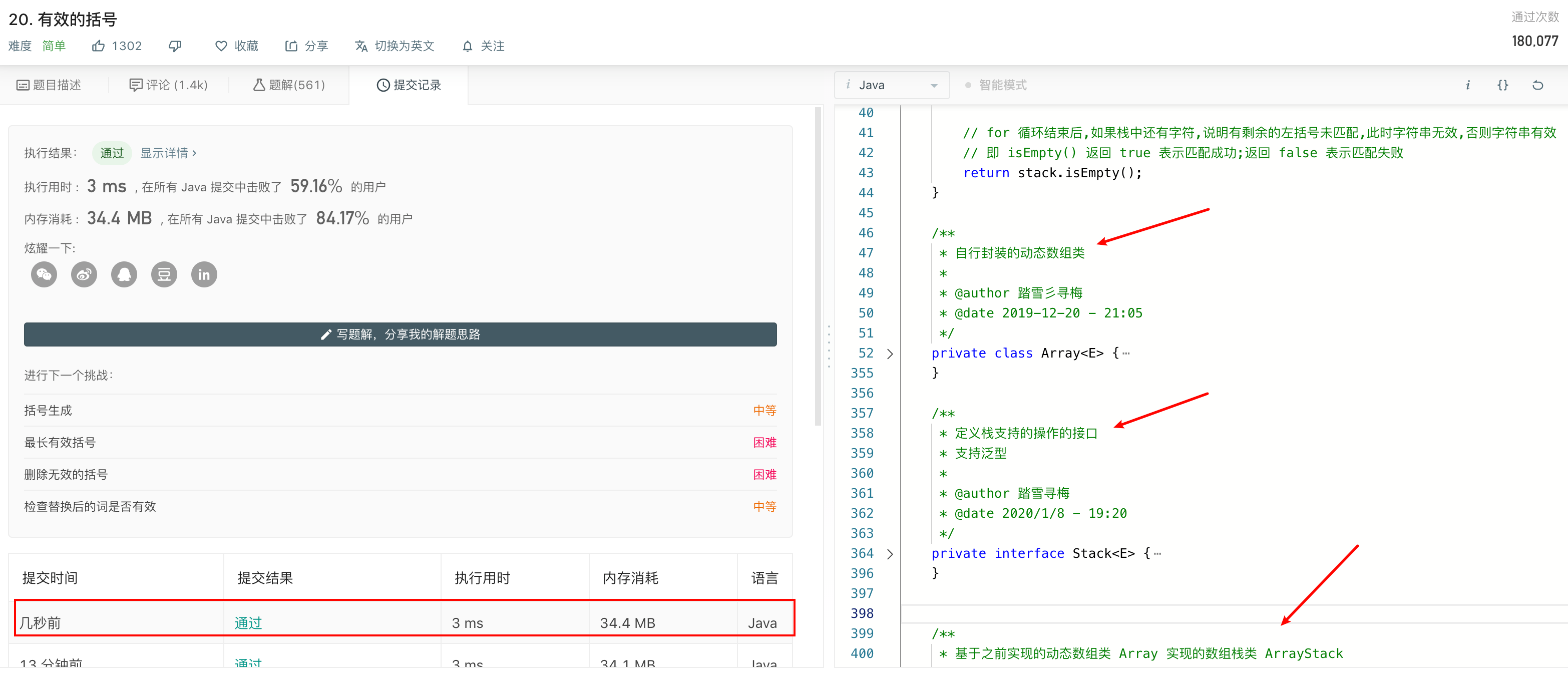## 队列的数据结构的实现

### 队列的基础知识回顾

1. 队列也是一种线性结构

2. 相比数组，队列对应的操作是数组的子集。

• 也就是说，队列是可基于数组实现的，可以将队列看成一个特殊的数组。
3. 队列只能从一端（队尾）添加元素，只能从另一端（队首）取出元素。

• 其实和现实生活中的排队差不多，队头的人离去，新来的人排在队尾。
4. 队列是一种先进先出 (FIFO：First In First Out) 的数据结构。

### 基于数组的队列的实现

1. 入队：void enqueue(E element)

2. 出队: E dequeue()

3. 查看队首元素: E getFront()

4. 获取队列元素个数: int getSize()

5. 判断队列是否为空: boolean isEmpty()

#### 具体代码设计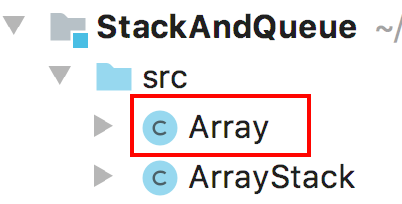Queue 接口类的实现:

/**
* 定义队列的基本操作的接口
* 支持泛型
*
* @author 踏雪寻梅
* @date 2020/1/9 - 16:52
*/
public interface Queue<E> {
/**
* 获取队列中元素个数
*
* @return 队列中如果有元素,返回队列中当前元素个数;队列中如果没有元素返回 0
*/
int getSize();

/**
* 判断队列是否为空
*
* @return 队列为空,返回 true;队列不为空,返回 false
*/
boolean isEmpty();

/**
* 入队
* 将元素 element 添加到队尾
*
* @param element 入队的元素
*/
void enqueue(E element);

/**
* 出队
* 将队首的元素出队并返回
*
* @return 返回当前出队的队首的元素
*/
E dequeue();

/**
* 查看当前队首元素
*
* @return 返回当前的队首元素
*/
E getFront();
}


ArrayQueue 类的实现:

ArrayQueue 类代码实现如下：

/**
* 基于之前实现的动态数组类 Array 实现的数组队列类 ArrayQueue
* 同样支持泛型
*
* @author 踏雪寻梅
* @date 2020/1/10 - 18:17
*/
public class ArrayQueue<E> implements Queue<E> {
/**
* 动态数组 array
* 基于 array 实现队列的操作
*/
private Array<E> array;

/**
* 构造函数
* 创建一个容量为 capacity 的数组队列
*
* @param capacity 要创建的队列的容量,由用户指定
*/
public ArrayQueue(int capacity) {
array = new Array<>(capacity);
}

/**
* 默认构造函数
* 创建一个默认容量的数组队列
*/
public ArrayQueue() {
array = new Array<>();
}

/**
* 获取队列的容量
* ArrayQueue 特有的方法
*
* @return 返回队列的容量
*/
public int getCapacity() {
// 复用 array 的 getCapacity() 方法即可
return array.getCapacity();
}

@Override
public int getSize() {
// 复用 array 的 getSize() 方法即可
return array.getSize();
}

@Override
public boolean isEmpty() {
// 复用 array 的 isEmpty() 方法即可
return array.isEmpty();
}

@Override
public void enqueue(E element) {
// 将数组的末尾作为队尾,复用 array 的 addLast() 方法实现
}

@Override
public E dequeue() {
// 将数组的首部作为队首,复用 array 的 removeFirst() 方法将队首元素出队并返回
return array.removeFirst();
}

@Override
public E getFront() {
// 复用 array 的 getFirst() 方法获取队首元素
return array.getFirst();
}

/**
* 重写 toString 方法返回数组队列的信息
*
* @return 返回数组队列的当前信息
*/
@Override
public String toString() {
StringBuilder result = new StringBuilder();
result.append("ArrayQueue: ");
result.append("size: ").append(array.getSize()).append(" ");
result.append("capacity: ").append(array.getCapacity()).append(" ");
result.append("front -> [ ");
for (int i = 0; i < array.getSize(); i++) {
result.append(array.get(i));
// 如果不是最后一个元素
if (i != array.getSize() - 1) {
result.append(", ");
}
}
result.append(" ] <- tail");
return result.toString();
}
}


/**
* 测试 ArrayQueue
*/
public static void main(String[] args) {
ArrayQueue<Integer> queue = new ArrayQueue<>();

// 判断队列是否为空
System.out.println("当前队列是否为空: " + queue.isEmpty());

for (int i = 0; i < 10; i++) {
// 入队
queue.enqueue(i);
// 显示入队过程
System.out.println(queue);

// 每入队 4 个元素就出队一次
if (i % 4 == 3) {
// 出队
queue.dequeue();
// 显示出队过程
System.out.println("\n" + queue + "\n");
}
}

// 判断队列是否为空
System.out.println("当前队列是否为空: " + queue.isEmpty());

// 获取队首元素
Integer front = queue.getFront();
System.out.println("当前队列队首元素为: " + front);
}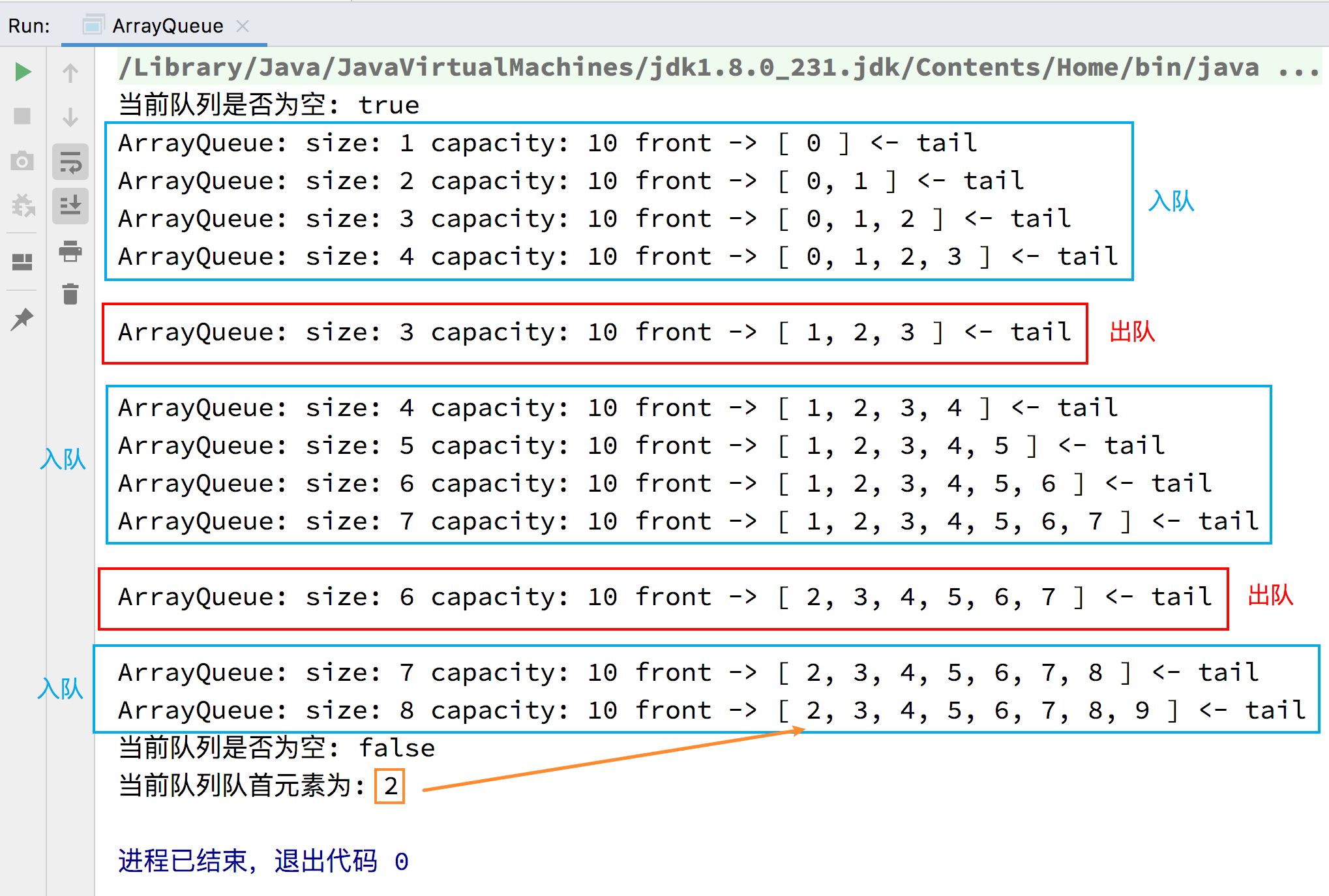#### 基于数组的队列的简单时间复杂度分析

• void enqueue(E element)：O(1) 均摊

• 复用了 array 的方法，所以可能触发 resize 方法进行伸缩容量，所以时间复杂度是均摊的。
• E dequeue()：O(n)

• 在出队的时候，当把队首的元素移出去之后，剩下的元素都要往前移动一个位置。

• 所以，对于当前实现的基于数组的队列，如果要放的数据量很大的话，比如 100 万、1000 万的数据量的时候，进行出队操作的时候时间性能消耗会很大。

• 对于这种情况，可使用循环队列来解决。
• E getFront()：O(1)

• int getSize()：O(1)

• boolean isEmpty()：O(1)

### 循环队列的实现1. 初始时，队列为空，front 和 tail 都指向 0，即 front == tail 表示队列为空，元素个数 size 的值也为 0，表示当前元素个数为 0。2. 当元素入队时，维护 tail 将其指向下一个元素入队时应该要放置的位置，即当前队尾元素的后一个位置。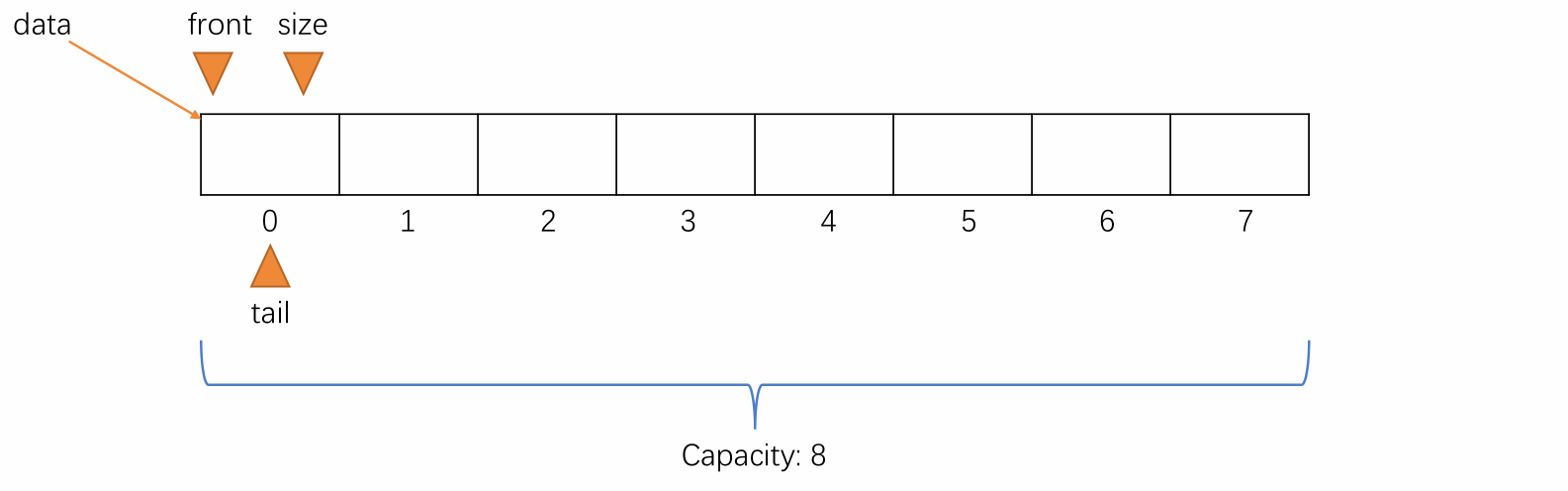3. 当元素出队时，维护 front 将其指向出队后的队列中的第一个元素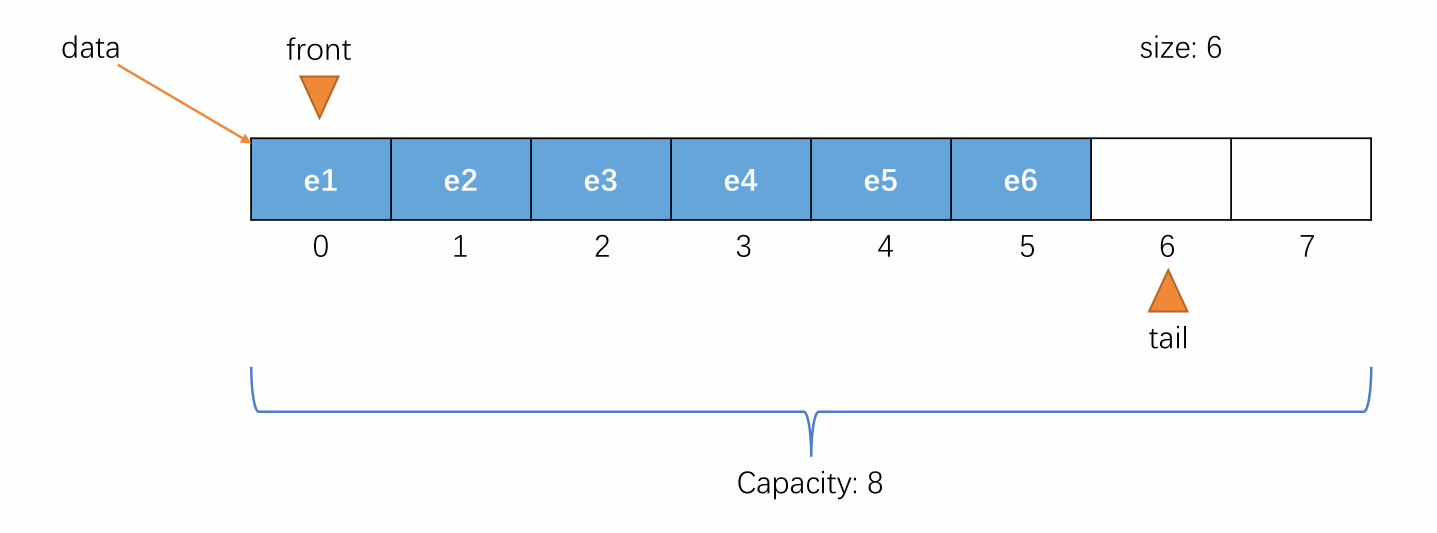4. 当元素入队到 tail 的值不能再加一且队列空间未满的时候，维护 tail 将其指向剩余空间中的第一个位置，使队列中的元素像处于一个环中一样进行循环

• 例如以下情况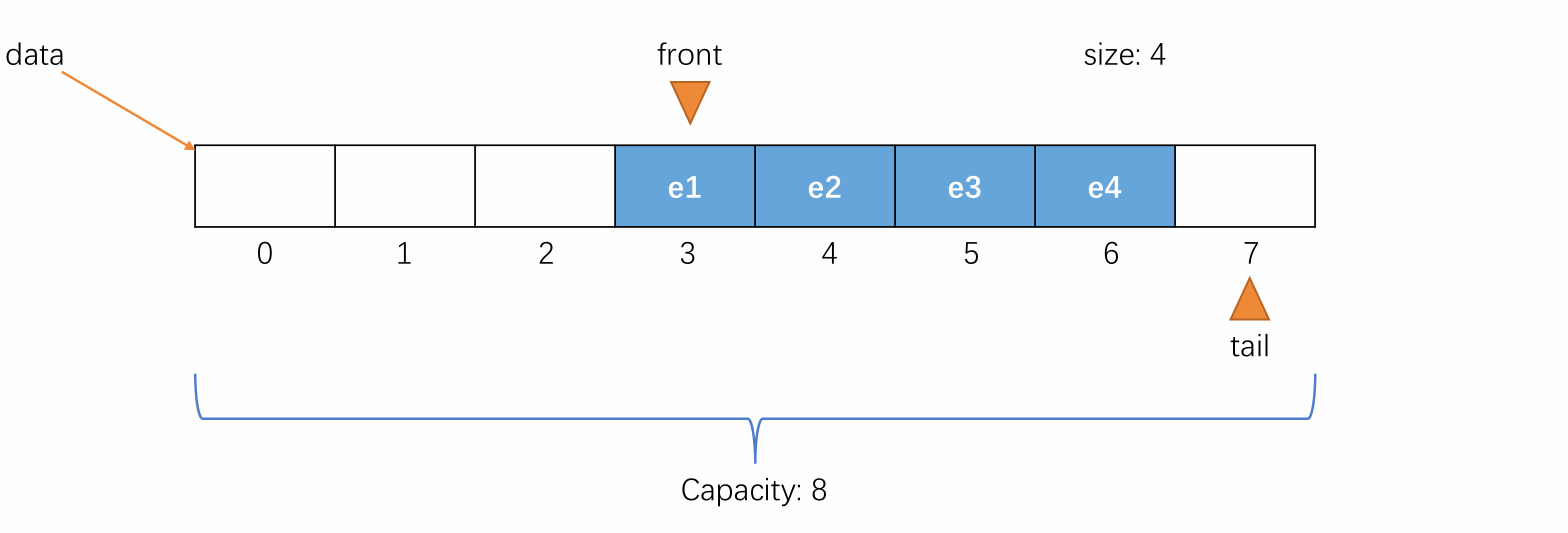• 此时可暂时得出元素入队时更改 tail 的值的公式：

(tail + 1) % capacity

• 当 tail 的值再加一就等于 front 的值时，此时队列还剩余一个空间此处这个剩余的空间设计为不记在队列容量中，是额外添加的），这时候表示队列为满，不能再入队，即 (tail + 1) % (capacity + 1) == front 表示队列满（capacity + 1 == data.length）

• 此时若再入队，就会让 front 和 tail 相等，由于front == tail 表示队列为空，此时队列又不为空，会产生矛盾。所以在循环队列中额外添加一个空间用来判断队列是否已满。具体过程如下图所示：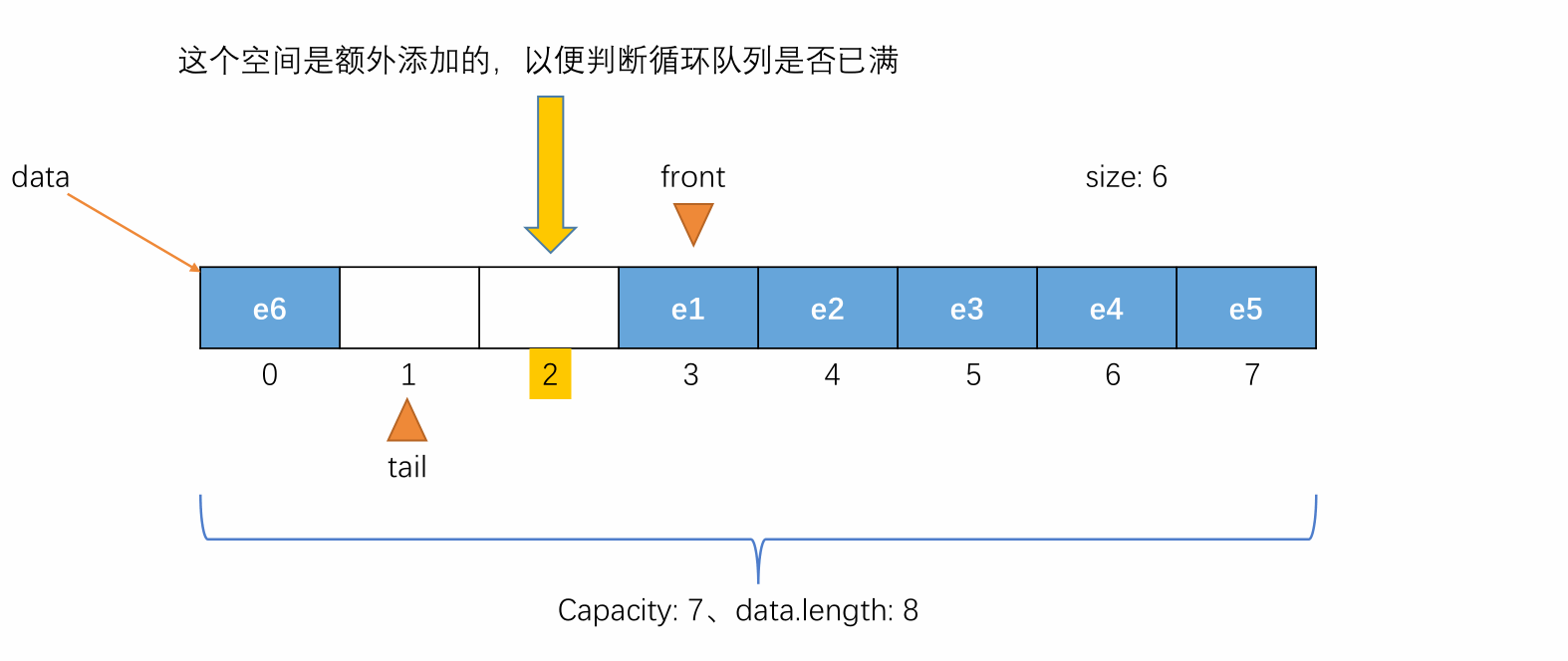• 综上可总结出关于循环队列的四个公式

1. front == tail 表示队列为空

2. (tail + 1) % data.length == front 表示队列为满

• 或 (tail + 1) % (capacity + 1) == front
3. 元素入队时更改 tail 的值的公式：(tail + 1) % data.length

• 因为已经设定了在队列中额外添加一个空间用于判断队列是否已满，所以更改 tail 时需要模的是队列底层数组的长度，而不是模队列的容量。可参照下图理解：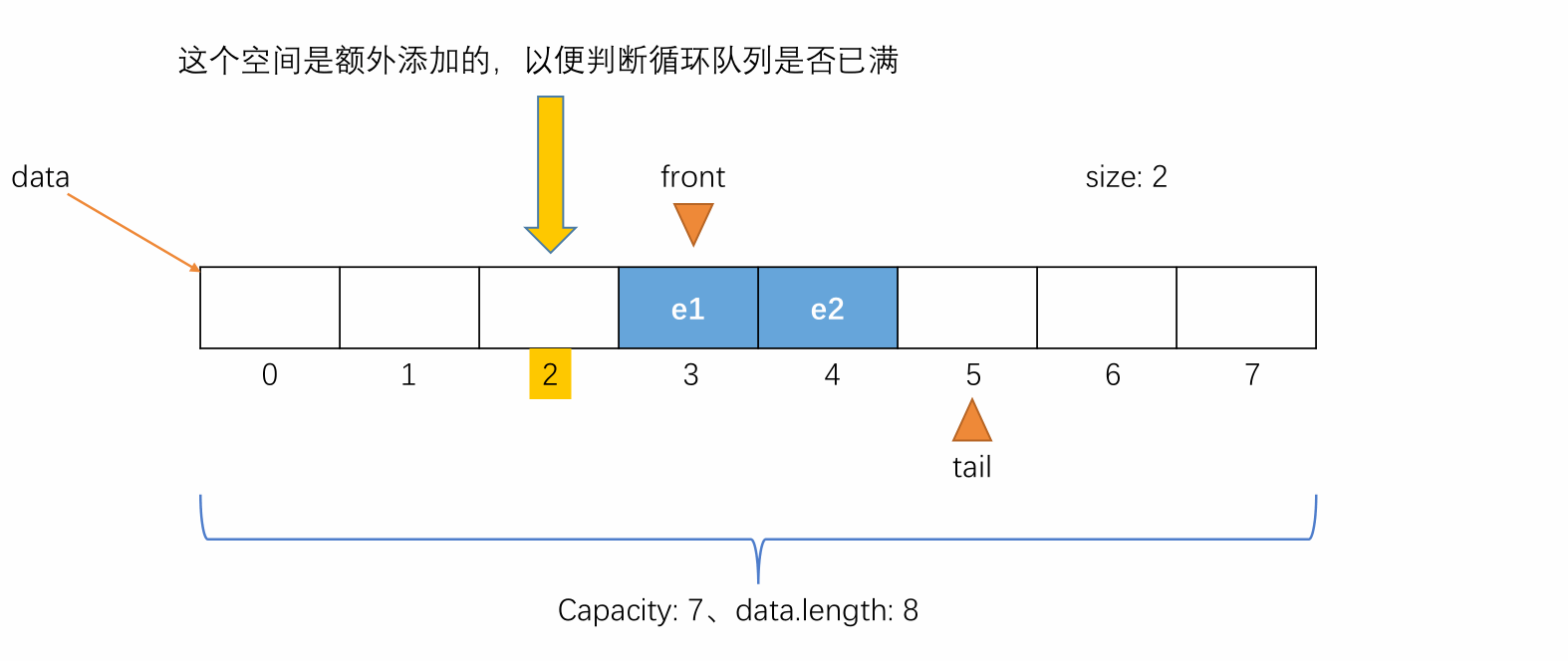4. 同理，可得出元素出队时更改 front 的值得公式：(front + 1) % data.length

#### 具体代码设计

/**
* 循环队列类 LoopQueue
* 支持泛型
*
* @author 踏雪寻梅
* @date 2020/1/10 - 21:35
*/
public class LoopQueue<E> implements Queue<E> {

/**
* 存放循环队列元素的底层数组
*/
private E[] data;

/**
* 队首索引
* 指向队列队首元素所在的索引
*/
private int front;

/**
* 队尾索引
* 指向新元素入队时应该要放置的索引
*/
private int tail;

/**
* 循环队列当前元素个数
*/
private int size;

/**
* 构造函数
* 构建一个容量为 capacity 的循环队列
*
* @param capacity 要创建的循环队列的容量,由用户指定
*/
public LoopQueue(int capacity) {
// 因为在循环队列中额外添加了一个空间用来判断队列是否已满,所以构建 data 时多加了一个空间
data = (E[]) new Object[capacity + 1];
// 循环队列初始化
front = 0;
tail = 0;
size = 0;
}

/**
* 默认构造函数
* 构建一个容量为 10 的循环队列
*/
public LoopQueue() {
this(10);
}

/**
* 获取循环队列的容量
*
* @return 返回循环队列的容量
*/
public int getCapacity() {
// 因为在循环队列中额外添加了一个空间用来判断队列是否已满,所以返回时将数组长度的值减一
return data.length - 1;
}

@Override
public int getSize() {
return size;
}

@Override
public boolean isEmpty() {
// front == tail 表示队列为空,返回 true;否则返回 false
return front == tail;
}

/**
* 将循环队列的容量更改为 newCapacity
*
* @param newCapacity 循环队列的新容量
*/
private void resize(int newCapacity) {
E[] newData = (E[]) new Object[newCapacity + 1];

// 将 data 的所有元素按顺序 (front ~ tail) 转移到 newData 的 [0, size - 1] 处
for (int i = 0; i < size; i++) {
// 在将元素转移到扩容后的空间时,两个数组的 i 的值不一定对应
// 所以取 data 的数据时需要将 i 进行偏移：(i + front) % data.length
newData[i] = data[(i + front) % data.length];
}
// 将 data 指向扩容后的队列
data = newData;
// 重新设定队首、队尾索引的值,因为上处将 data 的所有元素按顺序 (front ~ tail) 转移到 newData 的 [0, size - 1] 处
front = 0;
tail = size;
}

@Override
public void enqueue(E element) {
// 入队前先查看队列是否已满,data.length <==> getCapacity() + 1
if ((tail + 1) % data.length == front) {
// 队列满时进行扩容
resize(getCapacity() * 2);
}
// 入队
data[tail] = element;
// 维护 tail
tail = (tail + 1) % data.length;
// 维护 size
size++;
}

@Override
public E dequeue() {
// 出队前查看队列是否为空
if (isEmpty()) {
// 队列为空,抛出一个非法参数异常说明空队列不能出队
throw new IllegalArgumentException("Cannot dequeue from an empty queue.");
}

// 保存出队元素,以返回给用户
E dequeueElement = data[front];

// 出队
// 将原队首元素置空,防止对象游离
data[front] = null;
// 维护 front
front = (front + 1) % data.length;
size--;
// 当出队到队列元素个数少到一定程度时,进行减容
if (size == getCapacity() / 4 && getCapacity() / 2 != 0) {
resize(getCapacity() / 2);
}

// 返回出队元素给用户
return dequeueElement;
}

@Override
public E getFront() {
// 查看队首元素前查看队列是否为空
if (isEmpty()) {
// 队列为空,抛出一个非法参数异常说明队列是空队列
throw new IllegalArgumentException("Queue is empty.");
}

// 返回队首元素给用户
return data[front];
}

/**
* 重写 toString 方法返回循环队列的信息
*
* @return 返回循环队列的当前信息
*/
@Override
public String toString() {
StringBuilder result = new StringBuilder();
result.append(String.format("LoopQueue: size = %d, capacity = %d\n", size, getCapacity()));
result.append("front -> [ ");
for (int i = front; i != tail; i = (i + 1) % data.length) {
result.append(data[i]);
// 如果不是最后一个元素
if ((i + 1) % data.length != tail) {
result.append(", ");
}
}
result.append(" ] <- tail");
return result.toString();
}
}


/**
* 测试 LoopQueue
*/
public static void main(String[] args) {
LoopQueue<Integer> queue = new LoopQueue<>();

// 判断队列是否为空
System.out.println("当前队列是否为空: " + queue.isEmpty());

for (int i = 0; i < 10; i++) {
// 入队
queue.enqueue(i);
// 显示入队过程
System.out.println(queue);

// 每入队 3 个元素就出队一次
if (i % 3 == 2) {
// 出队
queue.dequeue();
// 显示出队过程
System.out.println("\n" + queue + "\n");
}
}

// 判断队列是否为空
System.out.println("当前队列是否为空: " + queue.isEmpty());

// 获取队首元素
Integer front = queue.getFront();
System.out.println("当前队列队首元素为: " + front);
}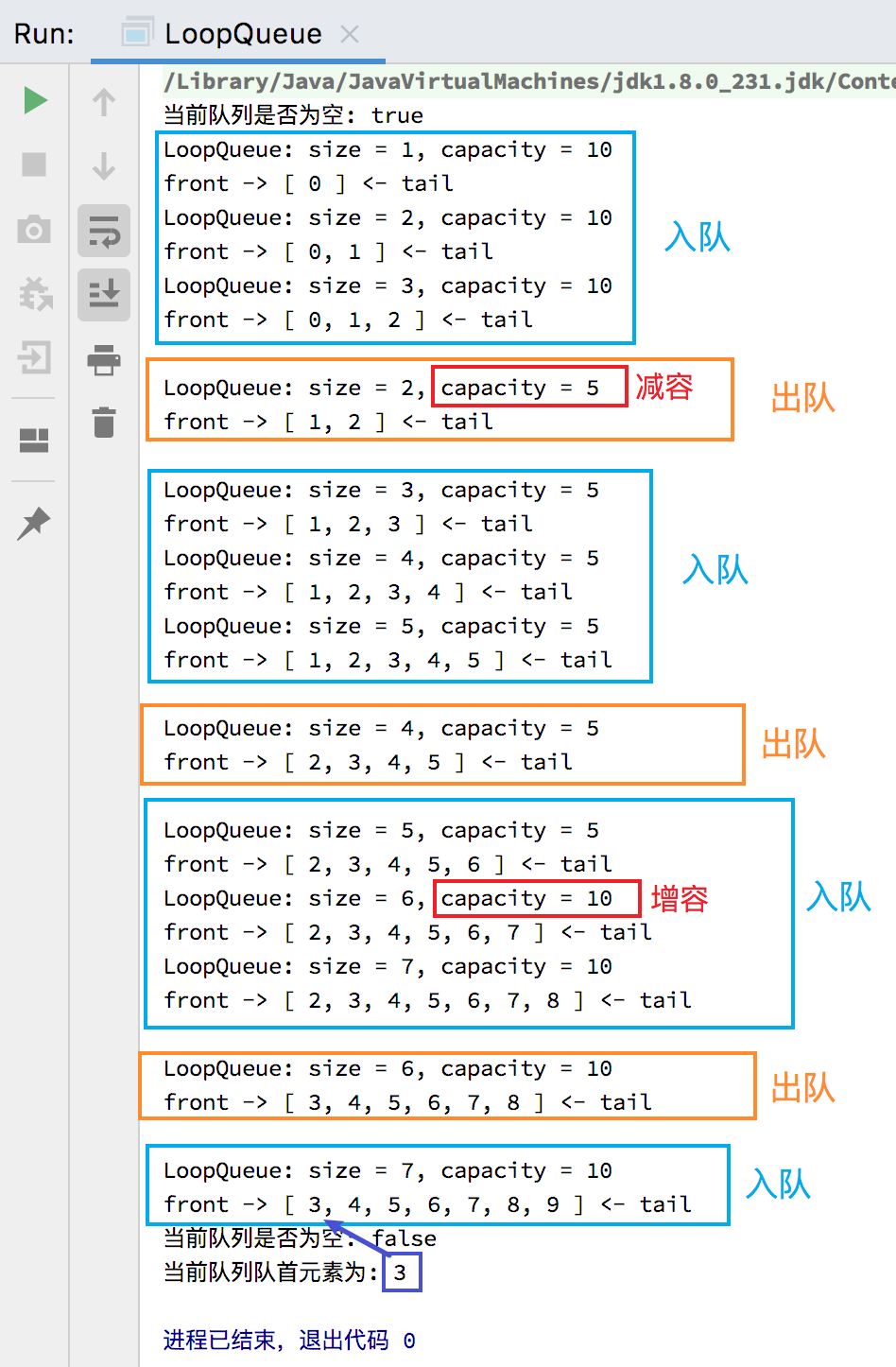#### 循环队列的简单时间复杂度分析

• void enqueue(E element)：O(1) 均摊

• E dequeue()：O(1) 均摊

• E getFront()：O(1)

• int getSize()：O(1)

• boolean isEmpty()：O(1)

### 数组队列和循环队列间的比较

import java.util.Random;

/**
* 测试 ArrayQueue 和 LoopQueue 的效率差距
*
* @author 踏雪寻梅
* @date 2020/1/8 - 16:49
*/
public class Main {
public static void main(String[] args) {
// 测试数据量
int opCount = 100000;

// 测试数组队列所需要的时间
ArrayQueue<Integer> arrayQueue = new ArrayQueue<>();
double arrayQueueTime = testQueue(arrayQueue, opCount);
System.out.println("arrayQueueTime: " + arrayQueueTime + " s.");

// 测试循环队列所需要的时间
LoopQueue<Integer> loopQueue = new LoopQueue<>();
double loopQueueTime = testQueue(loopQueue, opCount);
System.out.println("loopQueueTime: " + loopQueueTime + " s.");

// 计算两者间的差距
double multiple = arrayQueueTime / loopQueueTime;
System.out.println("在这台机器上,对于 " + opCount + " 的数据量,loopQueue 用时比 arrayQueue 用时大约快 " + multiple + " 倍.");
}

/**
* 测试使用队列 queue 运行 opCount 个 enqueue 和 dequeue 操作所需要的时间,单位: 秒
*
* @param queue 测试的队列
* @param opCount 测试的数据量
* @return 返回整个测试过程所需要的时间,单位: 秒
*/
private static double testQueue(Queue<Integer> queue, int opCount) {
long startTime = System.nanoTime();

// 用于生成随机数入队
Random random = new Random();

// opCount 次 enqueue
for (int i = 0; i < opCount; i++) {
// 入队
queue.enqueue(random.nextInt(Integer.MAX_VALUE));
}

// opCount 次 dequeue
for (int i = 0; i < opCount; i++) {
// 出队
queue.dequeue();
}

long endTime = System.nanoTime();

// 将纳秒单位的时间转换为秒单位
return (endTime - startTime) / 1000000000.0;
}
}


• 对于数组队列而言，入队时单次操作是 O(1) 的，而 opCount 次入队是 O(n) 的；出队时单次操作是 O(n) 的，而 opCount 次出队则是 O(n2) 的；所以对于整个 testQueue 方法，数组队列的时间复杂度是 O(n2) 级别的。

• 对于循环队列而言，入队时和出队时单次操作都是均摊复杂度 O(1) 的，所以 opCount 次入队和出队则是 O(n) 的，所以对于整个 testQueue 方法，循环队列的时间复杂度是 O(n) 级别的。

• 所以，可以预估：随着 n 的值越来越大，数组队列的时间消耗会比循环队列大许多。posted @ 2020-09-05 14:04  踏雪彡寻梅  阅读(753)  评论(0编辑  收藏  举报